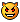# Startup Info Problem

## Recommended Posts

This is my first script using AutoIt and I am trying to pull as much Startup data as I can. The problem I am having when I run the script below is that the first couple of entries for \$StartData[\$LoopNum] are empty but the later ones contain the data. I'm using version 3.1.1.55. Can someone take a look at this and help me figure out where I'm going wrong?

Script:

#include <Array.au3>

#cs

\$StartData [x] = Name in Registry

\$StartData [x] = Name in Registry Value

\$StartData [x] = File Name

\$StartData [x] = File Path without flags

\$StartData [x] = File Version

\$StartData [x] = Description <-- Figure This Out Later

\$StartData [x] = Copyright <-- Figure This Out Later

\$StartData [x] = Registry Path

#ce

\$ProcessTotal = ProcessList()

\$RunLoop = \$ProcessTotal

Dim \$StartData[ (\$ProcessTotal) ]

Dim \$Var, \$RegVar

\$RegVar = ""

\$RegVar = "HKLM\Software\Microsoft\Windows\CurrentVersion\Run"

\$RegVar = "HKLM\Software\Microsoft\Windows\CurrentVersion\RunOnce"

\$RegVar = "HKLM\Software\Microsoft\Windows\CurrentVersion\RunServices"

\$RegVar = "HKCU\Software\Microsoft\Windows\CurrentVersion\Run"

\$RegVar = "HKCU\Software\Microsoft\Windows\CurrentVersion\RunOnce"

\$LastLoop = 0

\$PathLoop = 0

For \$PathLoop = 1 To 5

\$RegPath = \$RegVar[\$PathLoop]

\$LoopNum = 0

Do

\$LoopNum = \$LoopNum + 1

\$StartData[\$LoopNum] = RegEnumVal(\$RegPath, \$LoopNum)

If \$StartData[\$LoopNum] = "" Then

\$LoopNum = \$RunLoop

Else

\$len = StringLen(\$StartData[\$LoopNum])

\$baby = ""

\$BSTest = ""

\$STest = ""

\$RFTest = ""

For \$BSTest = \$len To 1 Step - 1

\$yeah = StringMid(\$StartData[\$LoopNum], \$BSTest, 1)

If \$BSTest = 1 Then \$baby = \$StartData[\$LoopNum]

If \$yeah = "\" Then

\$baby = StringMid(\$StartData[\$LoopNum], (\$BSTest + 1))

\$FirstBaby = StringMid(\$StartData[\$LoopNum], 1, \$BSTest)

\$BSTest = 1

EndIf

Next

If StringRight(\$baby, 1) = '"' Then \$baby = StringTrimRight(\$baby, 1)

For \$STest = \$len To 1 Step - 1

\$yeah2 = StringMid(\$baby, \$STest, 1)

If \$STest = 1 Then \$StartData[\$LoopNum] = \$baby

If \$yeah2 = " " Then

\$StartData[\$LoopNum] = StringMid(\$baby, 1, (\$STest - 1))

\$STest = 1

EndIf

Next

If StringRight(\$StartData[\$LoopNum], 1) = '"' Then \$StartData[\$LoopNum] = StringTrimRight(\$StartData[\$LoopNum], 1)

\$StartData[\$LoopNum] = \$FirstBaby & \$StartData[\$LoopNum]

If StringLeft(\$StartData[\$LoopNum], 1) = '"' Then \$StartData[\$LoopNum] = StringTrimLeft(\$StartData[\$LoopNum], 1)

\$StartData[\$LoopNum] = "FileVersion: " & FileGetVersion(\$StartData[\$LoopNum])

\$StartData[\$LoopNum] = "Description: "

\$StartData[\$LoopNum] = \$RegPath

\$LastLoop = \$LastLoop +1

EndIf

Until \$LoopNum = \$RunLoop

Next

\$PathLoop = 0

\$LoopRun = 0

\$Var = ""

For \$LoopRun = 1 To (\$LastLoop - 1)

; MsgBox(0, "Test", \$Var)

\$Var = \$Var & \$StartData[\$LoopRun] & ", " & \$StartData[\$LoopRun] & ", " & \$StartData[\$LoopRun] & ", " & \$StartData[\$LoopRun] & @CRLF & \$StartData[\$LoopRun] & ", " & \$StartData[\$LoopRun] & ", " & \$StartData[\$LoopRun] & ", " & \$StartData[\$LoopRun] & @CRLF & @CRLF

Next

MsgBox(0, "Startup", \$Var)

Not All Who Wander Are Lost
##### Share on other sites

Did a little test, only ran this against one reg section.

3 of the 4 were in the message box.

checked against the process' running and 3 of the 4 were there.

Is this what you expect?

Also when I ran the whole thing and then just the one section, whole, some were missing the name, one section all info was there for same keys.

Don't argue with an idiot; people watching may not be able to tell the difference.

##### Share on other sites

My goal for the script is to find all the data loading in the Run, RunOnce, etc. registry keys and load it into an array. Once I can accomplish this, I figured I would write in some functions to manipulate that data. I used the ProcessList() function to get a max limit for the array. When I run the script, it finds all 9 items I have in those keys, it's just those first few keys have the name missing, as you pointed out. Why it is working only a portion of the time is what confuses me.With this being my first script, what I'm trying to figure out is why the name is missing and how to correct it. I've looked all through the help file and don't seem to be coming up with the solution.

Any assistance you can provide in accomplishing this with AutoIt would be greatly appreciated.Not All Who Wander Are Lost
##### Share on other sites

err.. this is just my idea.. but

hijack this does an awsome job of this (if i recall it correctly)

and you can output it to a txt file and that txt file could be very very easily parsed...

but thats just what i would do, I am by farfoolish in many things tho## Create an account

Register a new account

• ### Recently Browsing   0 members

×

• Wiki

• Back

• #### Beta

• Git
• FAQ
• Our Picks
×
• Create New...